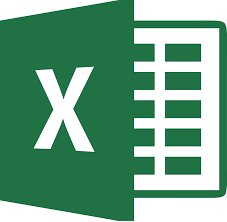# K2's Mastering Advanced Excel Functions and Formulas

• \$79.00

Course Description

Excel has more than 300 functions, many of which are unused by most accounting and finance professionals. Some of these little used functions can be used to calculate depreciation, mortgage interest and principal reduction, cash flow analysis over unequal periods, and trap errors. It's time for you to break out of your Excel cocoon. This two-hour session will put you on the path to improved Excel productivity by introducing you to powerful and time saving but little used Excel functions. Among other topics, you will learn about logical and conditional formulas, including using Boolean operators; advanced financial formulas such as XNPV and XIRR; innovative uses for Excel's date functions, and SUMPRODUCT, GETPIVOTDATA, and INDIRECT.

Major Topics

• Making advanced calculations with Excel's financial functions
• Creating calculations that are dependent on a condition or conditions
• Using array formulas to make calculations on a range of items
• Building date and time calculations
• Using GETPIVOTDATA to extract summarized data from a PivotTable
• Employing INDIRECT to modify range references in formulas

Learning Objectives

Upon completing this course, you should be able to:

• Use Excel's financial functions to compute depreciation, calculate mortgage interest and principal reduction, and perform conventional cash flow analysis
• List three Excel functions for making conditional calculations that can evaluate multiple conditions
• Explain the general process of entering an array formula in Excel
• Describe the benefits of using GETPIVOTDATA for extracting summarized data from a PivotTable

Who Should Attend: Accountants and finance professionals who want to become more productive in using Excel

Course Level: Intermediate

CPE Credits: 2 Hours Computer Software and Applications

Prerequisites: Good working knowledge of Excel